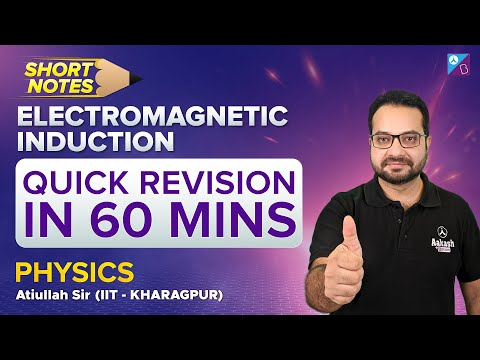# Electromagnetic Induction

## What Is Electromagnetic Induction?

Electromagnetic Induction was discovered by Michael Faraday in 1831, and James Clerk Maxwell mathematically described it as Faraday’s law of induction.

Electromagnetic Induction is a current produced because of voltage production (electromotive force) due to a changing magnetic field.

This either happens when a conductor is placed in a moving magnetic field (when using an AC power source) or when a conductor is constantly moving in a stationary magnetic field.

As per the setup given below, Michael Faraday arranged a conducting wire attached to a device to measure the voltage across the circuit. When a bar magnet is moved through the coiling, the voltage detector measures the voltage in the circuit.Through his experiment, he discovered that there are certain factors that influence this voltage production. They are:

1. Number of Coils: The induced voltage is directly proportional to the number of turns/coils of the wire. Greater the number of turns, greater is voltage produced
2. Changing Magnetic Field: Changing magnetic field affects the induced voltage. This can be done by either moving the magnetic field around the conductor or moving the conductor in the magnetic field.

You may also want to check out these concept related to induction:

## Watch the video and solve the NCERT exercise in the chapter Magnetic Effects of Electric Current Class 10## Applications of Electromagnetic Induction

Based on his experiments we now have Faraday’s law according to which the amount of voltage induced in a coil is proportional to the number of turns of the coil and the rate of changing magnetic field.

• AC generators work on the principle of electromagnetic induction.
• The working of electrical transformers are based on electromagnetic induction.
• The magnetic flow meter is based on electromagnetic induction.

### Electromagnetic Induction Formula

Mathematically, the induced voltage can be given by the following relation:

 e = N × dΦ / dt

Where,

• e is the induced voltage (in volts)
• N is the number of turns in the coil
• Φ is the magnetic flux – the amount of magnetic field at a surface (in Webbers)
• t is the time (in seconds)

The significance of this discovery is a way of producing electrical energy in a circuit by using magnetic fields and not just batteries. Everyday machines like motors, generators and transformers work on the principle of electromagnetic induction.

## The video is a rapid revision of electromagnetic induction in physics for JEE Main, presented by Atiullah Sir through short notes.Did you know that the principle of electromagnetic induction is also involved in working a credit card strip when you swipe it across the card machine? How do you think this works? [Hint: The strip is magnetic]

Q1

### Define Electromagnetic Induction.

Electromagnetic Induction is a current produced because of voltage production (electromotive force) due to a changing magnetic field.
Q2

### Who discovered Electromagnetic Induction?

Electromagnetic Induction was discovered by Michael Faraday in 1831.
Q3

### What is the significance of Electromagnetic Induction?

The significance of this discovery is a way of producing electrical energy in a circuit by using magnetic fields and not just batteries.
Q4

### Which machines work on the principle of Electromagnetic Induction?

Everyday machines like motors, generators and transformers work on the principle of electromagnetic induction.
Q5

### What are the applications of Electromagnetic Induction?

• AC generators work on the principle of electromagnetic induction.
• The working of electrical transformers are based on electromagnetic induction.
• The magnetic flow meter is based on electromagnetic induction.

## The below videos help to revise the chapter Magnetic Effects of Electric Current Class 10Stay tuned with BYJU’S to learn more Physics concepts with the help of interactive video lessons.

Test Your Knowledge On Electromagnetic Induction!